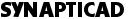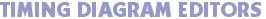# Waveform and Label Equations

Our tools provide the ability to generate signal waveforms from Temporal Equations and automatically label those waveforms using State Label equations. These features augment the drawing environment, and provide a quick way to generate signals without having to draw each individual signal transition. They also provide a way to generate signals that have precise edge placement.

#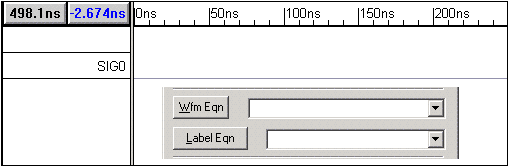## Temporal Equations

Temporal equations provide a quick way to generate signals that have a known pattern. Below is an example of a waveform equation that will generate 200 waveform segments, alternating in length between 50 and 100 ns.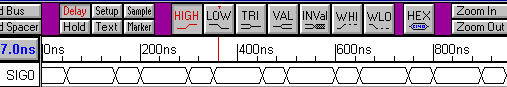Waveform Equation: (50 =V 100 = V)*100
Generate a 50 ns Valid transition, followed by 100 ns Valid transition, repeat 100 times.

• A Waveform Equation is a list of space-separated, time value pairs in the form: Time_value[units]=State_value (e.g. 10ns=Z).
• Valid State_values are: 0, 1 Z, V, H, L and I (capital i). The 0/1 are strong low and high, the H/L are weak high and low.
• For loops, enclose a list of time-value pairs in parenthesis and use the multiply symbol '*' followed by the number of times the list is to be repeated.

## Label Equation Functions

WaveFormer's Label equations are a great way to quickly create/edit complex stimulus vectors. Label equations generate state values on a signal's segments. Below are some examples of waveforms that can be created using the combination of Waveform (temporal) equations to set edge transition times and Label equations to set state values. Once you start using Label equations, you will find that you will be able to rapidly generate waveforms that would take hours to create by normal means.

## Generate a Series of Random State ValuesLabel Equation: RandInt(80,255)
Generates an array of 80 random integers within a range of 0 to 255 (inclusive).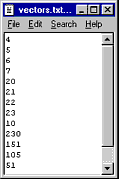## Import States from an Existing Signal

This is an easy way to import state values generated in a program such as MatLab®, Mathmatica®, or a user-written C program.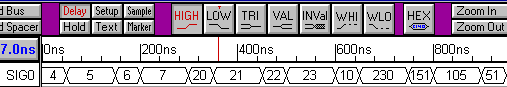Label Equation: File('vectors.txt')
Import a set of state values from the file vectors.txt.

## Copy States from an Existing Signal

The Signal function generates an array of states from an existing signal. It takes one optional argument, the name of the existing signal. If no signal name is specified, the states of the signal being operated on are used (this is particularly useful for incrementally executing a series of label functions if you want to see the results at each stage).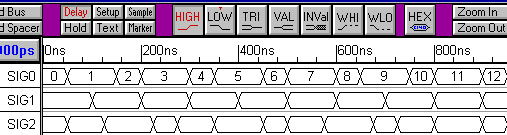Label Equation: Signal("SIG0")
Copying states from a signal named SIG0 to this signal.

## Perform function on every state

The map function allows you to perform a set of operations on every state in a state array. The first argument to the map function is the set of operations to perform, enclosed in brackets. The second argument is the array whose elements are to be operated on. The special variable \$_ is used to represent the state value to operate on (it takes on the value of each state in the array).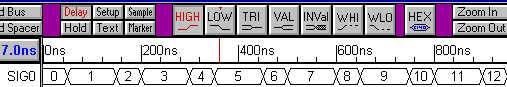Label Equation: map { \$_ + 5 } Signal()
The Signal function returns an array of the states on the current signal, and the map function increments each state in the array by 5.

Once you get familiar with it, the map function in combination with standard Perl language operations gives you extraordinary expressive power. Below is an equation that generates a set of values that can be displayed as a sine wave using the analog display capability: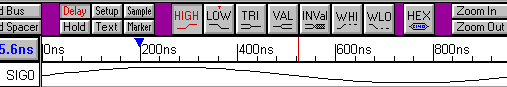Label Equation: map { sin(\$_) } Range(0,2*3.14159,100)
The Range function generates an array of 100 values between 0 and 2 PI radians. The map function computes the sine value for each element in this array. This technique is useful for generating analog waveforms.

## Create Your Own Label Equation Functions

One of the most powerful and easy to use capabilities of label equations is the ability to add your own user-defined functions to the list of available label equation functions. You do have to know a little bit of Perl, but it is easy to pick up if you have never worked with it. Engineers usually like Perl because you can quickly write code that does a lot. Perl combines a C-like syntax with standard Unix shell script capabilities.

 Phone: 540-953-3390 | Email: Sales Office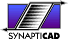COPYRIGHT 2019, SYNAPTICAD SALES, INC., ALL RIGHTS RESERVED.